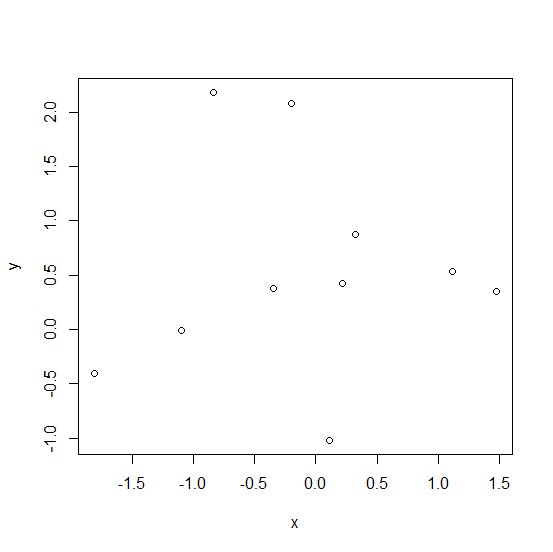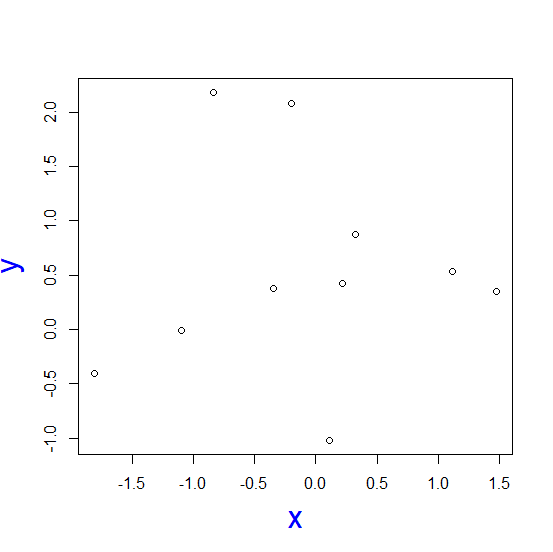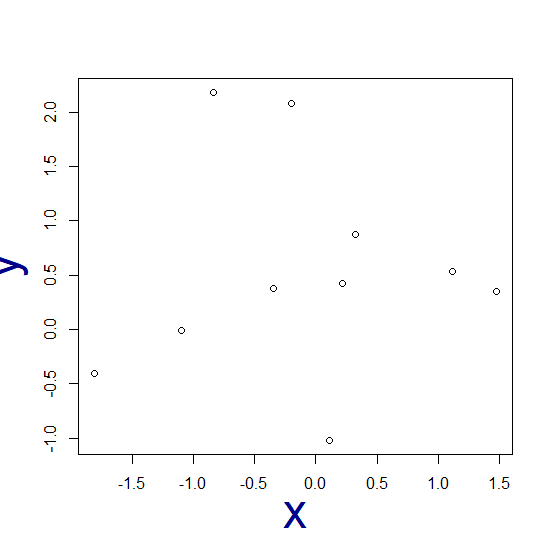# How to change the color and size of the axes labels of a plot created by using plot function in R?

The default size of axes labels created by using plot function does not seem to be large enough and also it does not look appealing. Therefore, we might want to change their size and color because the appearance of a plot matters a lot. This can be done by setting colors with col.lab and size with cex.lab.

## Example

Live Demo

> x<-rnorm(10)
> y<-rnorm(10,0.5)
> plot(x,y)

## OutputChanging the color of axes labels and the size of those axes labels −

> plot(x,y,col.lab="blue",cex.lab=2)

## Output> plot(x,y,col.lab="dark blue",cex.lab=3)

### Output